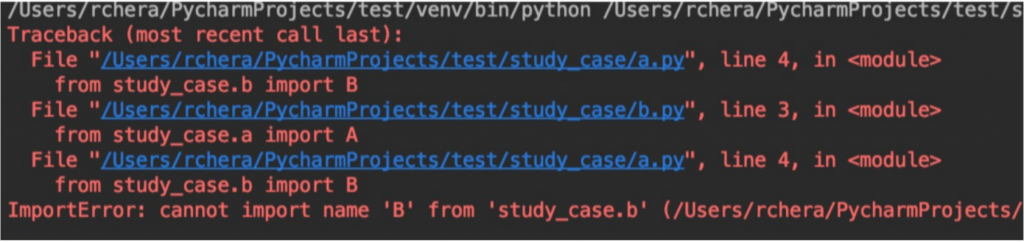• 欢迎访问DBA的辛酸事儿，推荐使用最新版火狐浏览器和Chrome浏览器访问本网站
• 欢迎大家关注博主公众号：DBA的辛酸事儿
• 博文中若有错误的地方，请大家指正，大家的指正是我前进的动力

# Python:关于py文件之间相互import的问题

2年前 (2021-11-15) 899次浏览

https://www.zhihu.com/question/19887316

https://blog.igevin.info/posts/how-to-avoid-python-circle-import-error/

PS：大神都建议不要在python中交叉循环引用（即不要在a中调用b，然后b中又调用a），最好规划好代码的层级，哪些是公共方法，哪些是业务逻辑，把这些提前想好，有利于后期的维护～

OK，接下来按照网上给出的方法，用一个例子演示下如何解决python中循环引用的问题实例演示

a.py

```from study_case.b import B

class A:
@staticmethod
def a1(number):
return number * number

@staticmethod
def a2():
value = B().b(1)
return value

if __name__ == '__main__':
t = A()
print(t.a1(2))
```

b.py

```from study_case.a import A

class B:
@staticmethod
def b(x):
value = A().a1(1)
return value + x

if __name__ == '__main__':
t = B()
print(t.b(1))

``````import study_case.a

class B:
@staticmethod
def b(x):
value = study_case.a.A().a1(1)
return value + x

if __name__ == '__main__':
t = B()
print(t.b(1))
```

```from study_case import a

class B:
@staticmethod
def b(x):
value = a.A().a1(1)
return value + x

if __name__ == '__main__':
t = B()
print(t.b(1))
```

```class B:
@staticmethod
def b(x):
from study_case.a import A
value = A().a1(1)
return value + x

if __name__ == '__main__':
t = B()
print(t.b(1))
```• 版权声明

本站的文章和资源来自互联网或者站长
的原创，按照 CC BY -NC -SA 3.0 CN
协议发布和共享，转载或引用本站文章
应遵循相同协议。如果有侵犯版权的资
源请尽快联系站长，我们会在24h内删
除有争议的资源。
• 网站导航

• 推荐阅读

• 关于本站